Next: Fun with Visualizing Modular Up: The Shafarevich-Tate Group Previous: Verifying the Full Conjecture   Contents

## Finiteness of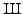A major very central conjecture in modern number theory is that if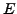is an elliptic curve over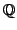then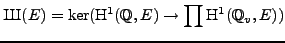is finite. This is a theorem when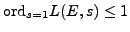, and is not known in a single case when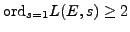. Proving finiteness offor any curve of rank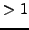would be a massively important result that would have huge ramifications. Much work toward the Birch and Swinnerton-Dyer conjecture (of Greenberg, Skinner, Urban, Nekovar, etc.) assumes finiteness of. Note that if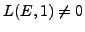or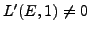, then it is a theorem that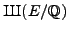is finite; there isn't even a single curve with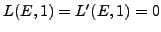for which finiteness of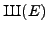is known.

As far as I can tell nobody has even the slightest clue how to prove this. However, we can at least try to do some computations.

Problem 8.8.5   Letbe the elliptic curve defined by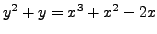of conductor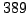. This curve has rank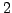.
1. Verify that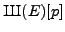is finite for 5 primes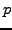.
2. Verify thatis finite for all primes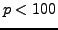.

Remark 8.8.6 (From Christian Wuthrich.)   Seems doable. I quickly run shark up to p = 53, it does not take too long. As far as I remember I never actually checked if shark is optimal when computing the p-adic L-function.

Remark 8.8.7   In theory one can verify that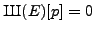for anyusing a-descent. In practice this does not seem practical except for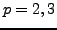. For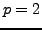use mwrank or simon_two_descent in SAGE. For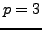use three_selmer_rank in SAGE (this command just calls MAGMA and runs code of Michael Stoll).

Remark 8.8.8   The work of Cristian Wuthrich and Stein mentioned in Section 8.8.1 could be used to verify finiteness for many. And Perrin-Riou does exactly this in the supersingular case in [PR03]. (In fact, she does much more, in that she computes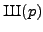in the whole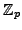tower. Shark contains now her computations with a few modifications. - from Christian Wuthrich.)Next: Fun with Visualizing Modular Up: The Shafarevich-Tate Group Previous: Verifying the Full Conjecture   Contents
William Stein 2006-10-20